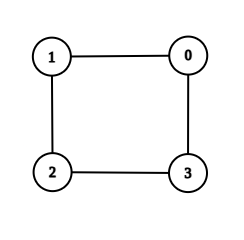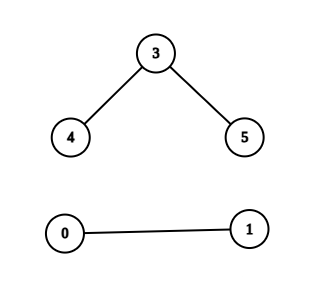0

# Get DFS Path

Difficulty: EASYContributed By
Avg. time to solve
15 min
Success Rate
90%

Problem Statement

#### Find the path using DFS and print the first path that you encountered.

##### Note:
``````Vertices are numbered through 0 to V-1.
``````
##### Input Format :
``````The first line contains a single integer ‘T’ denoting the number of test cases. Then each test case follows.

The first line of each test case contains two integers ‘V’ and ‘E’ denoting the number of vertices and edges in the graph.

The next ‘E’ lines of the test case contain two space-separated integers ‘a’ and ‘b’ denoting that there exists an edge between ‘a’ and ‘b’.

The last line of the test case contains two space-separated integers ‘v1’ and ‘v2’ denoting the starting vertex and ending vertex.
``````
##### Output Format :
``````For each test case, print the path from ‘v1’ to ‘v2’ in reverse order.

Output for each test case will be printed in a separate line.
``````
##### Note :
``````You are not required to print anything; it has already been taken care of. Just implement the function and return a list of paths.

If there is no path between the vertices return an empty list.If the path is valid then it will print true else it will print false.
``````
##### Constraints :
``````1 <= T <= 10
1 <= V <= 1000
1 <= E <= (V * (V - 1)) / 2
0 <= v1, v2 <= V-1

Time Limit: 1sec
``````
##### Sample Input 1 :
``````2
4 4
0 1
0 3
1 2
2 3
1 3
6 3
5 3
0 1
3 4
0 3
``````
##### Sample Output 1 :
``````true
false
``````
##### Explanation For Sample Output 1 :``````In the first test case, there are two paths from 1 to 3. I.e. 1 -> 2 -> 3 or 1 -> 0 -> 3. So you can print any one of them.
````````````In the second test case, there is no path from 0 to 3. Hence return an empty string.
``````
##### Sample Input 2 :
``````2
2 1
0 1
1 0
5 4
0 1
1 2
2 3
3 4
0 4
``````
##### Sample Output 2 :
``````true
true
``````Console